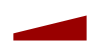Reflexxes Motion Libraries  Manual and Documentation (Type II, Version 1.2.6)
Description of Input Values

The input values of the position-based On-Line Trajectory Generation (OTG) algorithm are set-up through the classes RMLPositionInputParameters and RMLPositionFlags, and the input values of the velocity-based On-Line Trajectory Generation algorithm are set-up through the classes RMLVelocityInputParameters and RMLVelocityFlags. For a detailed description of these classes, please refer to the class documentation, and for a description of the output values, please refer to the Description of Output Values.

## Input Values for the Position-based On-Line Trajectory Generation AlgorithmInput and output values of the position-based Type II On-Line Trajectory Generation algorithm of the Reflexxes Motion Libraries.

The position-based Type II On-Line Trajectory Generation algorithm is executed by a call of ReflexxesAPI::RMLPosition(). The input values for the algorithm at a time instant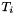are contained in RMLPositionInputParameters:

Each vector contains the values for all degrees of freedom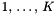, for instance,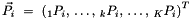such that the current position value of the degree of freedom with the index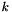is represented by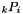.

The selection vector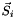can be used to mask degrees of freedom individually. If the value of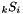is false, the degree of freedom with the indexwill not be considered by the algorithm.

Besides the input values RMLPositionInputParameters, the class RMLPositionFlags can be used to determine and obtain a certain behavior. The following flags may be used:

A first and simple example that shows, how the position-based input values are commonly set-up, please refer to the Example 1 — Introduction to the Position-based algorithm. A description of the output values of this example can be found at the page Description of Output Values. Please also refer to the Section Input Requirements for Numerical Stability, which describes the input domains of the algorithm.

Note:
The variables

are only used by the Type IV Reflexxes Motion Library.

RMLPositionInputParameters
RMLPositionFlags
Description of Output Values

## Input Values for the Velocity-based On-Line Trajectory Generation Algorithm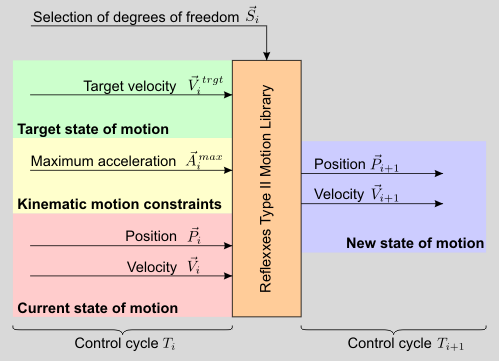Input and output values of the velocity-based Type II On-Line Trajectory Generation algorithm of the Reflexxes Motion Libraries.

The velocity-based Type II On-Line Trajectory Generation algorithm is executed by a call of ReflexxesAPI::RMLVelocity(), and its input values are the very same as for the position-based algorithm (see above), but the values for the target position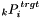and the maximum velocity vector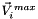are not considered, and the flag RMLPositionFlags::KeepCurrentVelocityInCaseOfFallbackStrategy is not available.

The input values for the velocity-based algorithm at a time instantare contained in RMLVelocityInputParameters:

Besides the input values RMLVelocityInputParameters, the class RMLVelocityFlags can be used to determine and obtain a certain behavior. The following flags may be used:

A first and simple example that shows, how the velocity-based input values are commonly set-up, please refer to the Example 4 — Introduction to the Velocity-based algorithm. A description of the output values of this example can be found at the page Description of Output Values. Please also refer to the Section Input Requirements for Numerical Stability, which describes the input domains of the algorithm.

Note:
The variables

are only used by the Type IV Reflexxes Motion Library.

Note:
The velocity-based algorithm is also used in the second layer of the safety mechanism to guarantee valid output value for any given set of input values (cf. Safety: Three Layers of Error Handling).

RMLVelocityInputParameters
RMLVelocityFlags
Description of Output Values

## Input Requirements for Numerical Stability

In order to assure feasibility and numerical stability, two basic conditions have to be fulfilled for each selected degree of freedomin order to obtain correct output values:

1. The maximum velocity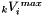and the maximum acceleration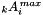must be greater than zero:

•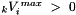•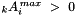2. For the position-based algorithm, the elements of the maximum velocity vectorhas to be greater or equal to the elements of the target velocity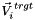.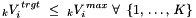3. All input values have to be within a certain range of magnitude. For the position-based algorithm this is range is specified by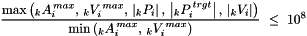and for the velocity-based algorithm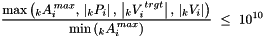Note:
1. Although this condition looks like a limitation, in real-world systems it does not lead to a disadvantage, because the range of eight orders of magnitude should be very sufficient for any application. As the velocity-based algorithm is much simpler from a numerical point of view, ten orders of magnitude are allowed here.

2. If this condition is not fulfilled, the Type II Reflexxes Motion Library will try to provide correct output values, and in many cases this will be possible, but no guarantee can be given. Valid output values that lead to steady, continuous and jerk-limited motion trajectories are guaranteed in any case (cf. Safety: Three Layers of Error Handling).

Example for a set of valid input values for one degree of freedomat time instantused for the position-based algorithm:Reason: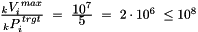.

Example for a set of invalid input values for one degree of freedomat time instantused for the position-based algorithm:Reason: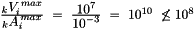.

The check, whether the input values are valid, can be done with the methods RMLPositionInputParameters::CheckForValidity() and RMLVelocityInputParameters::CheckForValidity().

User documentation of the Reflexxes Motion Libraries by Reflexxes GmbH (Company Information, Impressum). This document was generated with Doxygen on Mon Jul 7 2014 13:21:09. Copyright 2010–2014.